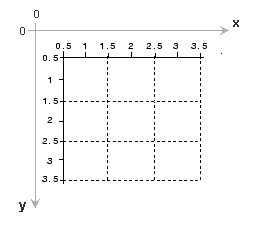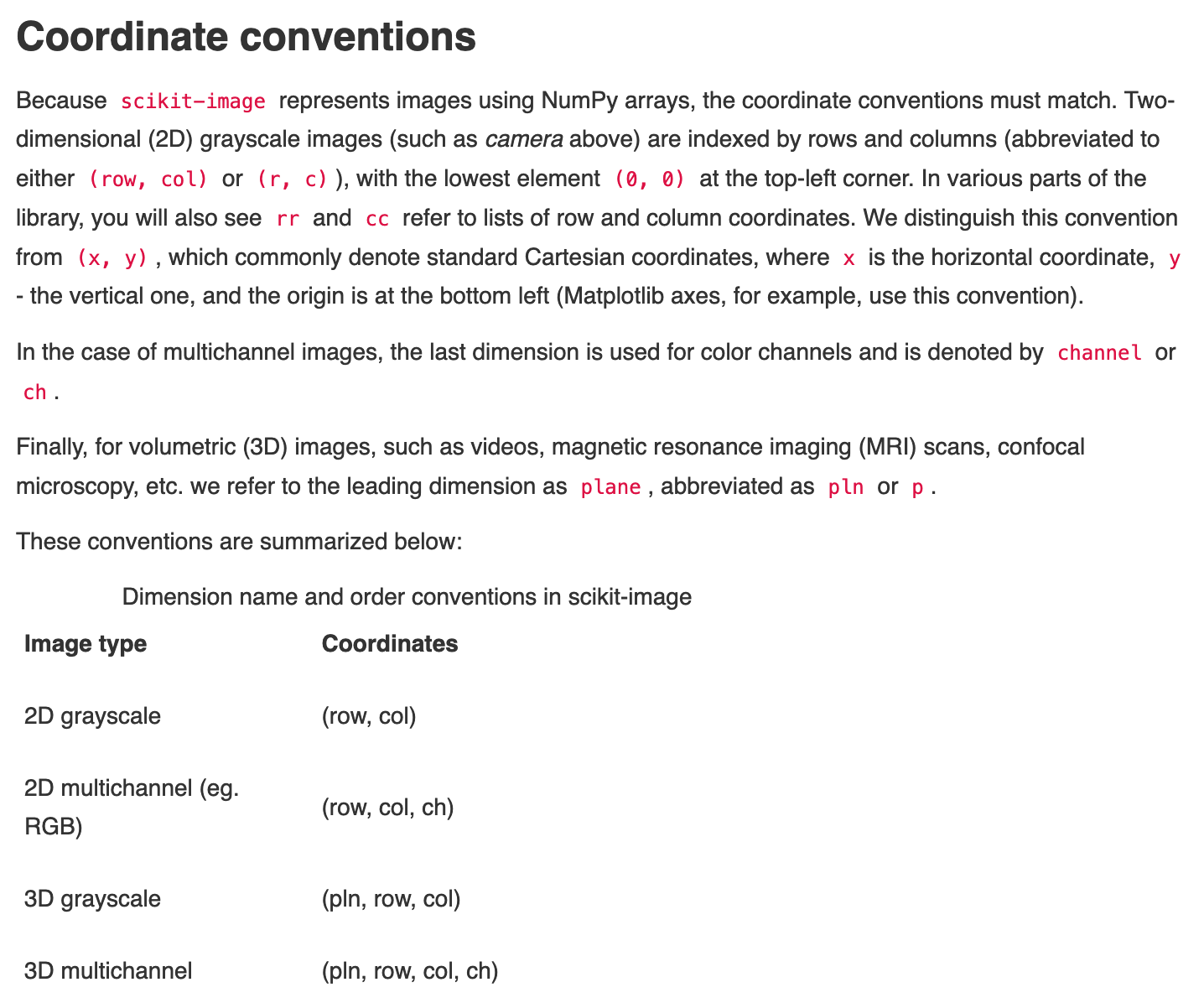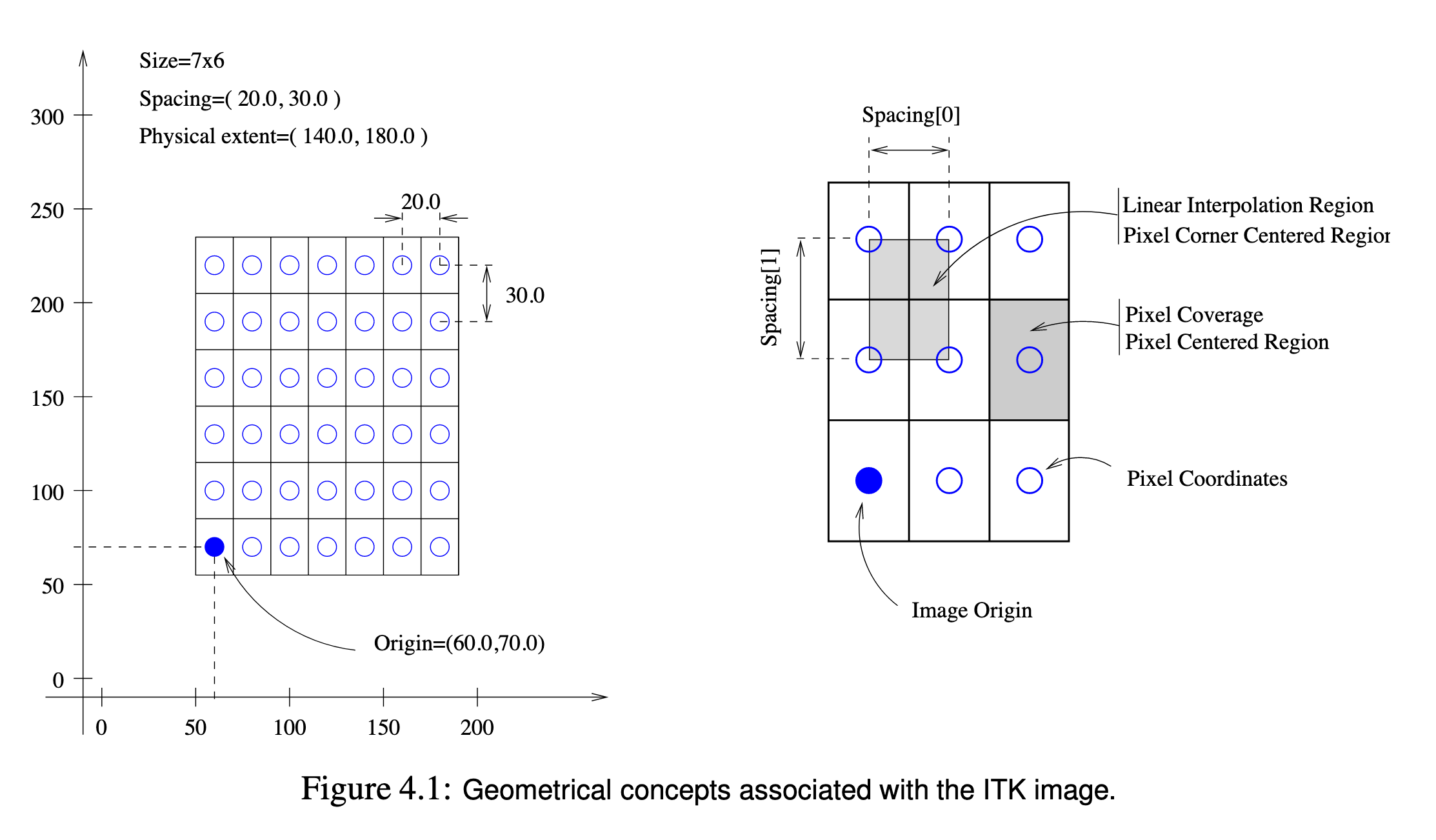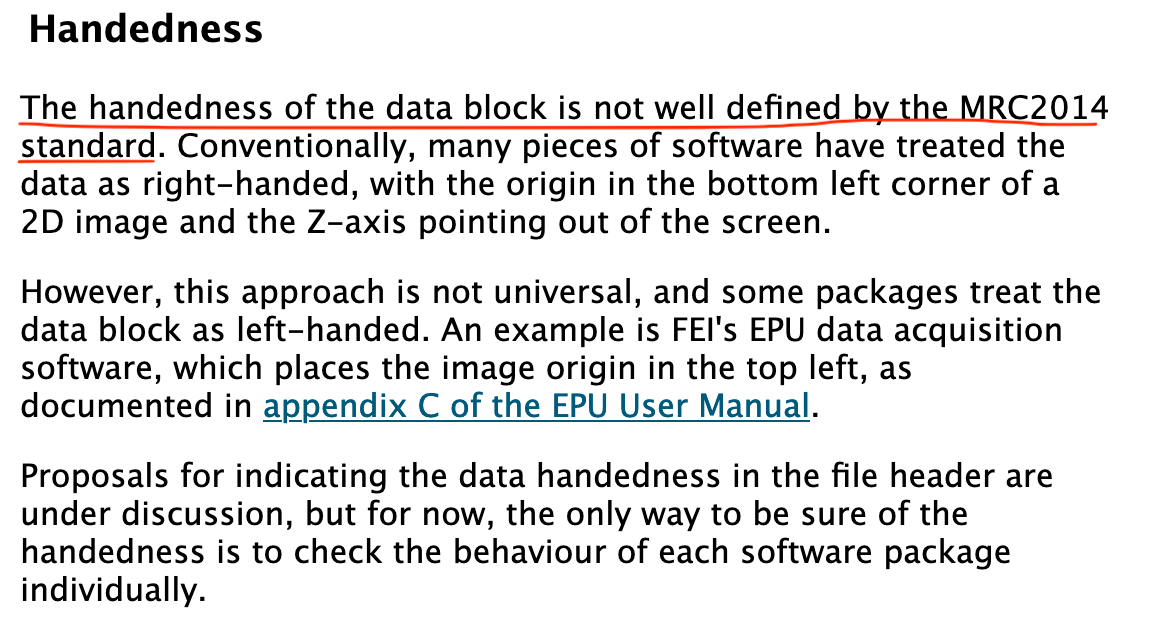# On Working with 3D Data

Posted 1 year, 7 months ago | Originally written on 13 Oct 2021

The ideas are straightforward; the problem is how these ideas are expressed through various programming APIs.

The cast of characters: space handedness, image coordinate systems

Discrete vs continuous spaces

Domain issues: MRC files

## Granularity

Consider an arbitrary 3D dataset. We rarely think of 3D data apart from some space and the most natural space is the cartesian space consisting of the dimensions x, y and z. This not only takes advantage of our considerable famiarity with the cartesian coordinate system but easily lends itself to both continous and discrete representations: the discrete case would simply involve define evenly spaced voxels between any two physical points.

## Orientation/Handedness

We need to label the axes of this space. Unfortunately, we have two options: one having z axes jutting out perpendicular to the xy plane in one direction and the other having it jut out in the opposite direction. These two options provide the basis of handedness. Suppose we curl the finger of a hand so that the fingers began in the direction of increasing x then curled inwards towards the origin in the direction of decreasing y (a counterclockwise motion) if the thumb was pointing out of the xy plane (as would occur for the right hand) we call this axes orientation a right hand system. If we only swap the x and y axes then the opposite (a clockwise motion in the plane) with the z axis pointing outward (as would occur for the left hand) would constitute a left hand system.

Any space can only be one of the two: right or left hand.

So far we have taken care of the two most important attributes: orientation (right vs. left handed) and granularity (discrete vs. continuous).

Next, we turn the problem of addressing: how we refer to parts of the space. Again, the most natural way to do this is with the familiar notation of a triple of values. If the space is continuous, (x, y, z) will refer to the value at that point in the space. If the space is discretised then we use the indices (i, j, k). Related to the notion of addressing is the notion of the size of the space. Continous spaces have physical sizes so that the space would be described by a triple of lengths (Lx, Ly, Lz) while discrete spaces would have the number of discretisations in each dimension expressed using familiar triples: (Nx, Ny, Nz). Therefore, when addressing a continous space we would expect the value of x to be found along the length, while for a discrete space the index j would also have to be found along Ny.

## Origin

Notice that the last sentence is quite vague ("found along"); this is because there is one more aspect of a space that we haven't covered yet: the location of the origin. Having the orientation, granularity and size are not sufficient to locate a space. We need to know where this space sits relative to the origin of the coordinate system.

Again, we have two perspectives to this: the physical origin specifies a linear transform that should be applied to the space to align its local origin to some global origin. If this is (0, 0, 0), then the local and global origins are coincident. If it is some value (Ox, Oy, Oz) then we would need to subtract the corresponding vector to every point in our space to align our space with the global origin.

Additionally, the discrete origin specifies which voxel (volume element) has the index (i=0, j=0, z=0).

For simplicity, most applications will treat both origins as (x=0, y=0, z=0) and (i=0, j=0, k=0) making subsequent operations easy.

## World vs. Actors

With the fundamental properties of spaces out of the way it is now sensible to distinguish between the world and actors. The world is a space which is populated with various objects referred to as actors. Actors placed in the world are located relative to the world (or global) space i.e. coordinate system. Nevertheless, each actor has its own local coordinate system.

Alternatively, we can think of this as spaces interacting with each other.

## Where's the confusion, then?

### MATLABhttps://www.mathworks.com/help/images/intro_r_1.png

### `scikit-image`https://scikit-image.org/docs/stable/user_guide/numpy_images.html

### ITKhttps://itk.org/ItkSoftwareGuide.pdf

### `mrcfile`https://www.ccpem.ac.uk/mrc_format/mrc2014.php

## Sources of confusion

One of the main sources of confusion arises when images are treated as spaces. Conventionally, the directionality of an image is top-left to bottom-right in much the same way that text flows. This means that the first pixel corresponds to the first word and so on. When an image is treated as a space we have to coincide the image origin with the space origin and the latter wins. Effectively, the first voxel sits at the spatial origin. To add to the confusion, the lack of a handedness of the space means that 'reading direction' is ambiguous; it's bad enough in 2D but catastrophic in 3D. In 2D, we only have two axes so a simple transposition can resolve the issue. This is not the case in 3D as we have a total of six transpositions.